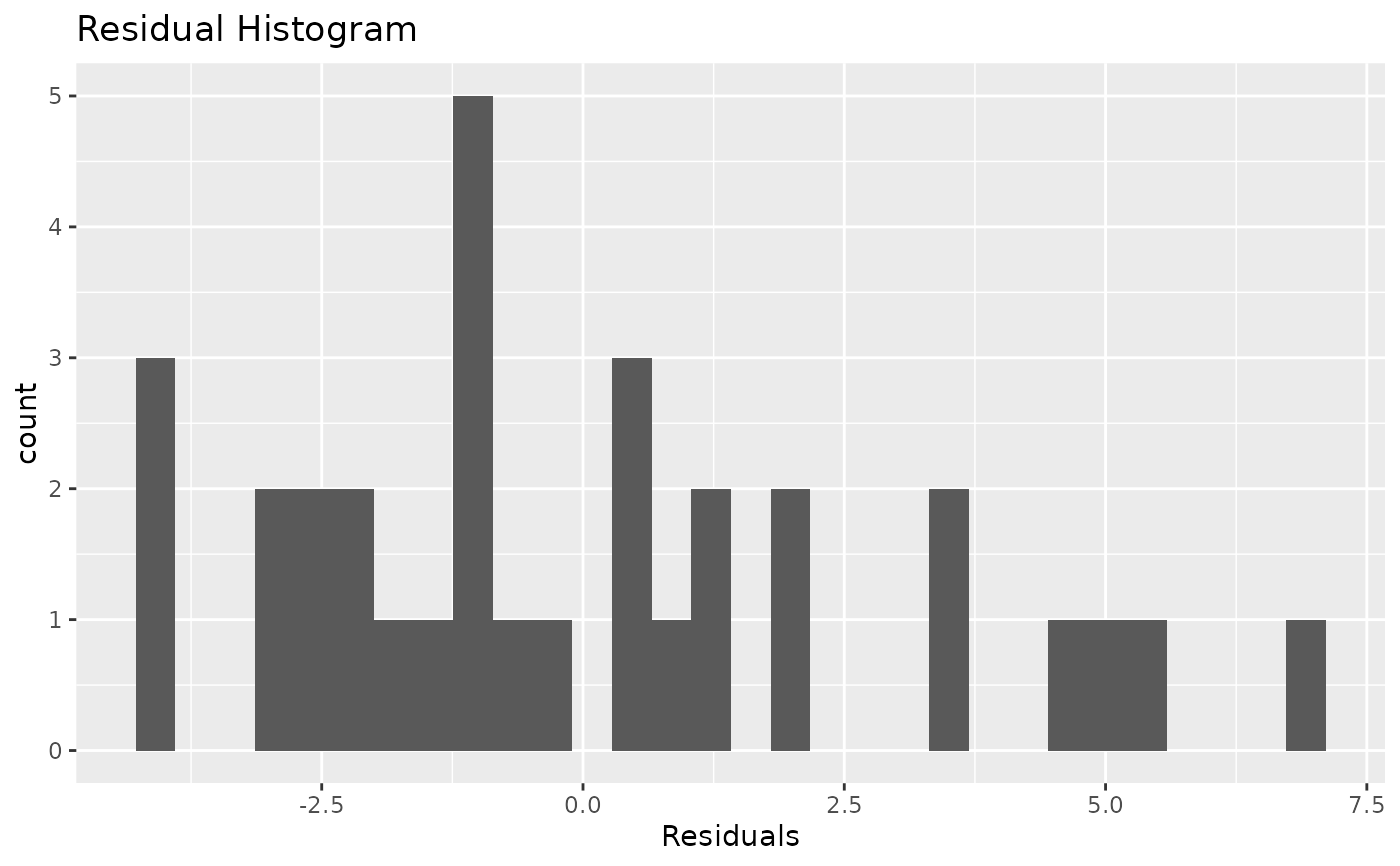Visualize the distribution of the residuals of a model.

stat_resid_hist(bins = 30, ...)

## Arguments

bins

...

Currently ignored. For extendability.

## Value

A ggplot2 layer for plotting a histogram of residuals.

## Examples

data(mtcars)
model <- lm(mpg ~ cyl + disp + hp, data = mtcars)
ggplot2::ggplot(data = model) + stat_resid_hist()